# For each of the following production functions calculate the ( MRTS v L,K ) a. Q...

For each of the following production functions calculate the ( MRTS v L,K )

a. Q = L^2/3 K^1/3 when Q=8

b. Q = 3L + K when Q=3

c. Q = min{3L, K} when Q=3

MRTSL,K = MPL/MPK.

MPL =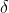Q/L.

MPK =Q/K.

MPL = 2/3*L-1/3 * K1/3.

MPL = 2/3 * (K/L)1/3

MPK = 1/3 * K-2/3 * L2/3

MPK = 1/3 * (L/K)2/3

MRTS = 0.5 * K/L.

b. MPL = 3

MPK = 1.

MRTS = 3/1 = 3.

c. MRTS = 0.

This is so because this production function represents perfect complements, the two inputs are used in fixed proportions. They are used together, and not substituted for each other.

#### Earn Coin

Coins can be redeemed for fabulous gifts.

Similar Homework Help Questions
• ### 3. For each of the following production functions, graph a typical isoquant and determine whether the marginal rate of...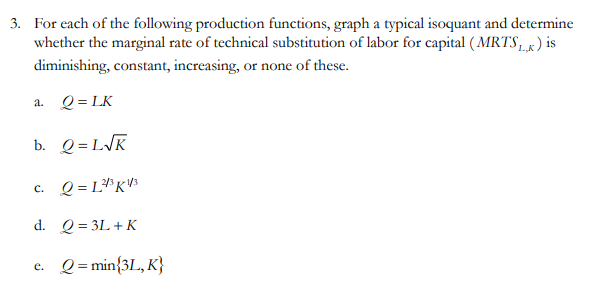3. For each of the following production functions, graph a typical isoquant and determine whether the marginal rate of technical substitution of labor for capital (MRTS ) is diminishing, constant, increasing, or none of these. a. Q=LK b. Q=LVK c. Q=L*K13 d. Q = 3L +K e. Q = min{3L, K} Show transcribed image text 3. For each of the following production functions, graph a typical isoquant and determine whether the marginal rate of technical substitution of labor for capital...

• ### 1. Below are production functions that turn capital (K) and labor (L) into output. For cach of th...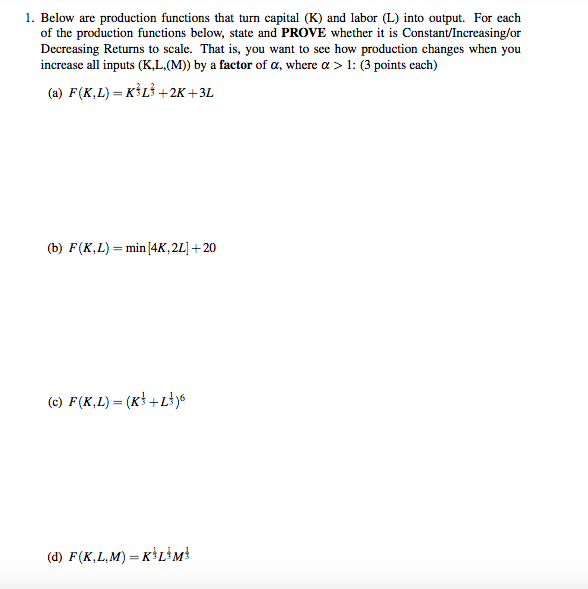1. Below are production functions that turn capital (K) and labor (L) into output. For cach of the production functions below, state and PROVE whether it is Constant/Increasing/or Decreasing Returns to scale. That is, you want to see how production changes when you increase all inputs (K,L, (M)) by a factor of a, where a > 1: (3 points each) (a) F(K,L)-KİLİ+2K +3L (b) F(K, L)=min/4K, 2L1+20 (d) F(K,L,M) KL3M 1. Below are production functions that turn capital (K) and...

• ### 4. Below are production functions that turn capital (K) and labor (L) into output. For each...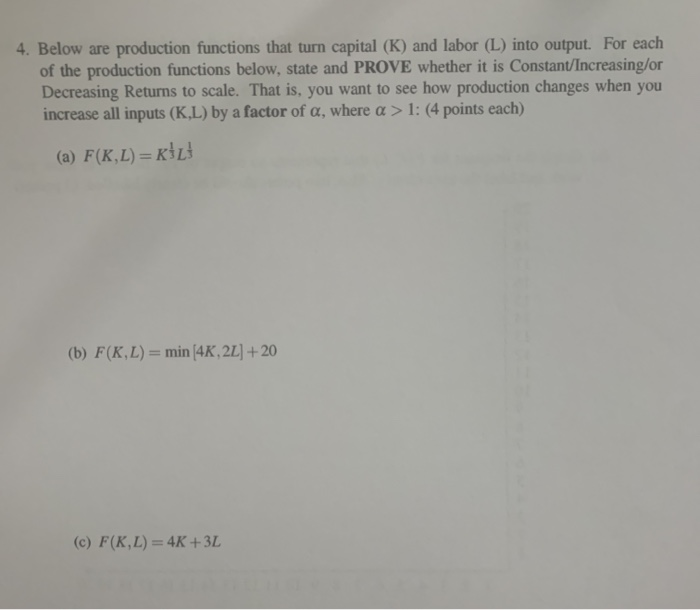4. Below are production functions that turn capital (K) and labor (L) into output. For each of the production functions below, state and PROVE whether it is Constant/Increasing/or Decreasing Returns to scale. That is, you want to see how production changes when you increase all inputs (K.L) by a factor of a, where a > 1: (4 points each) (a) F(K,L) =KİL (b) F(K,L) = min 4K, 2L] + 20 (c) F(K,L) = 4K +3L 5. For this problem you...

• ### Let's suppose that a firm's production technology is represented by the following production function q =...

Let's suppose that a firm's production technology is represented by the following production function q = F( K, L) = min { 2K, L } What is the MRTS when 2K > L a. Infinite b. 0 c. 1/2 d. 2 What is the MRTS when a. 0 b. 1/3 c. infinite d. 2

• ### For following two production functions: (1) Q = L1/4 K (2) Q = 2L + 4K...

For following two production functions: (1) Q = L1/4 K (2) Q = 2L + 4K a) Determine whether factor L is subject to diminishing marginal returns? What about K? b) Calculate the MRTS. c) Determine returns to scale: CRS, IRS or DRS. Show how you arrive at your answers. For function (2) also draw a few representative isoquants; fully label your graph.

• ### 1. Graph the short-run total product curves for each of the following production functions if K...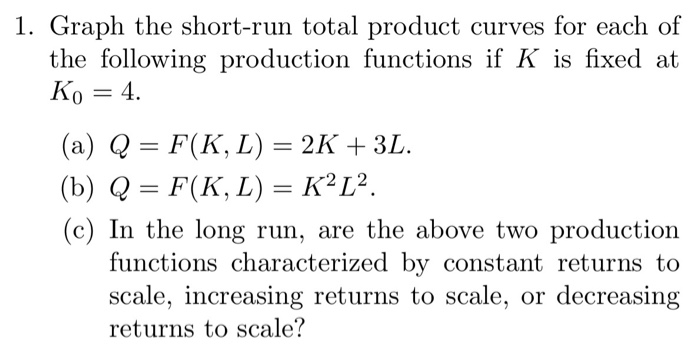1. Graph the short-run total product curves for each of the following production functions if K is fixed at Ko 4 (a) Q = F(K, L) = 2K + 3L. (b) Q = F(K, L) = K2L2. (c) In the long run, are the above two production functions characterized by constant returns to scale, increasing returns to scale, or decreasing returns to scale?

• ### Below are production functions that turn capital (K) and labor (L) into output. For each of...

Below are production functions that turn capital (K) and labor (L) into output. For each of the production functions below, state and PROVE whether it is Constant/Increasing/or Decreasing Returns to scale. That is, you want to see how production changes when you increase all inputs (K,L,(M)) by a factor of α, where α > 1: (4 points each) a) F(K,L) = K^1/3*L^1/3+2K+3 b) F(K,L) = sqr(K^3+L^3) c) F(K,L) = (K^2/4+L^2/4)^2 d) F(K,L,M) = min(K,L)*M

• ### 1. Below are production functions that turn capital (K) and labor (L) into output. For each...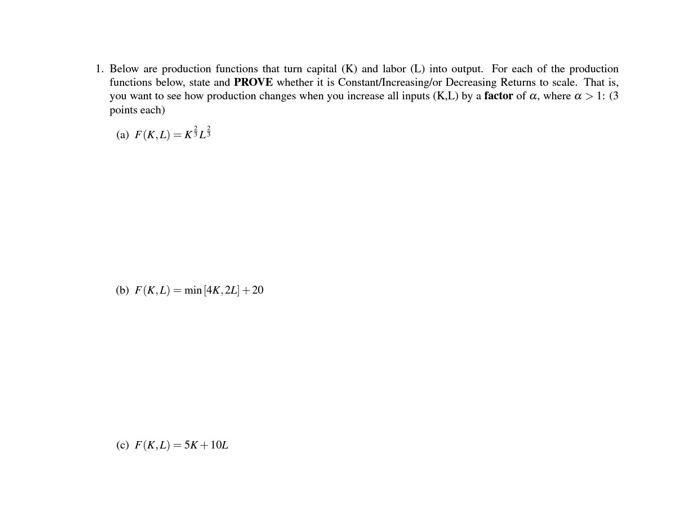1. Below are production functions that turn capital (K) and labor (L) into output. For each of the production functions below, state and PROVE whether it is Constant/Increasing/or Decreasing Returns to scale. That is, you want to see how production changes when you increase all inputs (K.L) by a factor of a, where a > 1: (3 points each) (a) F(KL)=KL (b) F(K,L) = min (4K, 22] + 20 (c) F(K,L) = 5K+10L

• ### W= Continuing to use the three production functions: q = h(K, L) = K(1/3) [(1/3), q=g(K,...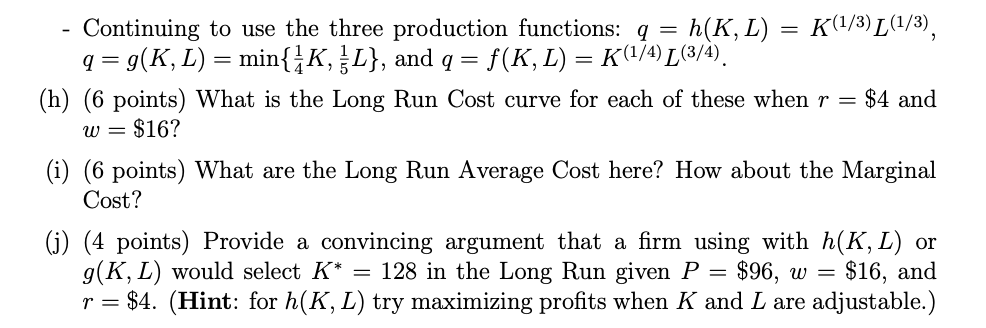W= Continuing to use the three production functions: q = h(K, L) = K(1/3) [(1/3), q=g(K, L) = min{įK, L}, and q = = f(K, L) = K (1/4) L (3/4). (h) (6 points) What is the Long Run Cost curve for each of these when r = $4 and$16? (i) (6 points) What are the Long Run Average Cost here? How about the Marginal Cost? (j) (4 points) Provide a convincing argument that a firm using with h(K,...

• ### 1. Below are production functions that turn capital (K) and labor (L) into output. For each...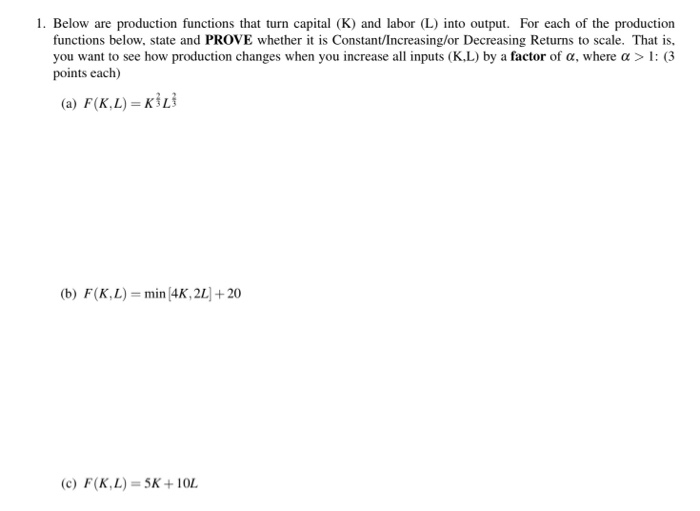1. Below are production functions that turn capital (K) and labor (L) into output. For each of the production functions below, state and PROVE whether it is Constant/Increasing/or Decreasing Returns to scale. That is, you want to see how production changes when you increase all inputs (KL) by a factor of a, where a > 1: (3 points each) (a) F(K.L) = (b) F(KL)= min (4K, 2L + 20 (c) F(K,L) = 5K+ 10L

Free Homework App